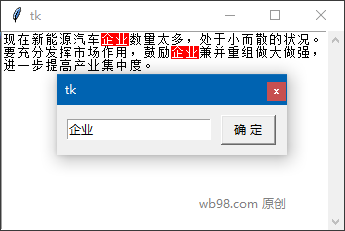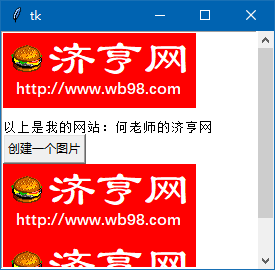# tkinter的Text组件的虚拟事件

### 虚拟事件：复制，剪切，粘贴

event_generate("<<Copy>>"))   # 虚拟复制事件

event_generate("<<Cut>>"))    # 虚拟剪切事件

event_generate("<<Paste>>"))  #虚拟粘贴事件

```from tkinter import *

popup.post(event.x_root,event.y_root) # 弹出右键菜单

root=Tk()

scr1=Scrollbar(root)  # 垂直滚动条
scr1.pack(side='right',fill=Y)

te1=Text(root)
te1.pack(side='left',fill=BOTH,expand=True)
te1.insert(END,'点击鼠标右键，测试一下复制，剪贴，粘贴功能。')

te1.config(yscrollcommand = scr1.set) # 滚动条的绑定
scr1.config(command = te1.yview)   # 滚动条的绑定

root.mainloop()```

运行结果### 关键词查找 search()方法

weizi=te1.search(关键词字符，开始位置，查找位置)

我在上面的代码里，在弹出菜单项再增加一个分割线，一个‘查找’菜单项。

```from tkinter import *

popup.post(event.x_root,event.y_root) # 弹出右键菜单
def findfrm(): # 显示查找窗口
top1=Toplevel(root,width=230,height=50) # 查找窗口

a,b=230,50 # 查找窗口的宽和高
c=root.winfo_x()+((root.winfo_width()-a)/2)
d=root.winfo_y()+((root.winfo_height()-b)/2)
top1.geometry('%dx%d+%d+%d' % (a,b,c,d)) # 查找窗口居中

top1.attributes("-toolwindow", 1) # 无最大化，最小化
top1.transient(root) # 窗口只置顶root之上
top1.resizable(False,False) # 不可调节窗体大小
top1.focus_force()  # 得到焦点

en1=Entry(top1)  # 输入查找关键字的单行文本框
but1=Button(top1,text="  确 定  ",command=lambda:findok(en1.get()))
en1.focus_force() # 单行文本框得到焦点
def findok(key): # 查找关键词函数
start='1.0' # 初始化，查找起始位置
te1.tag_remove('tag1','1.0',END) # 初始化，移除标签，但不删除标签的设置定义
if key=='': # 关键词为空，退出
return
while True: # 循环查找
weizi=te1.search(key,start,END)
print(weizi) # 输出查找返回的结果
if weizi=='' : # 返回值为空，就是找不到
break # 跳出循环
te1.tag_add('tag1',weizi, weizi+'+' + str(len(key))+'chars') # 找到了就新建一个标签
start=weizi+'+' + str(len(key))+'chars' # 找到了，就把起始位置定义为：找到位置+关键字的长度

root=Tk() # 源码来自wb98.com

scr1=Scrollbar(root)  # 垂直滚动条
scr1.pack(side='right',fill=Y)

te1=Text(root)
te1.pack(side='left',fill=BOTH,expand=True)

te1.config(yscrollcommand = scr1.set) # 滚动条的绑定
scr1.config(command = te1.yview)   # 滚动条的绑定

te1.tag_config('tag1',background='red',foreground='white') # 查找标签的设置定义：红底白字

root.mainloop()```

运行结果：```    while True: # 循环查找
weizi=te1.search(key,start,END)
print(weizi) # 输出查找返回的结果
if weizi=='' : # 返回值为空，就是找不到
break # 跳出循环
te1.tag_add('tag1',weizi, weizi+'+' + str(len(key))+'chars') # 找到了就新建一个标签
start=weizi+'+' + str(len(key))+'chars' # 找到了，就把起始位置定义为：找到位置+关键字的长度```

1 是为这个查找的关键字新建一个标签，这个标签的范围就是查找到的位置为起始位置，结束位置就是起始位置 + 关键字的长度。

2是把下一次查找的起始位置改成：查找到的位置+ 关键字的长度。

```    start='1.0' # 初始化，查找起始位置
te1.tag_remove('tag1','1.0',END) # 初始化，移除标签，但不删除标签的设置定义```

### Text组件插入图片，按钮组件

Text组件不但可以插入文本，也可以插入图片。

```from tkinter import *

root=Tk()

scr1=Scrollbar(root)  # 垂直滚动条
scr1.pack(side='right',fill=Y)

te1=Text(root,width=36,height=18)
te1.pack(side='left',fill=BOTH,expand=True)

img1=PhotoImage(file='wz.png')
te1.image_create(END,image=img1)

te1.insert(END,'\n\n以上是我的网站：何老师的济亨网')

te1.config(yscrollcommand = scr1.set) # 滚动条的绑定
scr1.config(command = te1.yview)   # 滚动条的绑定

root.mainloop()```Text组件还可以插入组件，这一点，是不是感觉Text组件有点框架组件的意思？

Text组件插入组件的方法是：先创建要插入的组件，然后用:

window_create(插入位置，window=组件)  插入组件。

Text组件插入的组件还可以触发相关事件，下面代码在上面的代码基础上再插入一个按钮。

```from tkinter import *

def okimg1():
te1.image_create(END,image=img1)

root=Tk()

scr1=Scrollbar(root)  # 垂直滚动条
scr1.pack(side='right',fill=Y)

te1=Text(root,width=36,height=18)
te1.pack(side='left',fill=BOTH,expand=True)

img1=PhotoImage(file='wz.png')
te1.image_create(END,image=img1)

te1.insert(END,'\n\n以上是我的网站：何老师的济亨网\n')

but1=Button(root,text="创建一个图片",command=okimg1) # 创建按钮
te1.window_create(END,window=but1) # 插入按钮

te1.config(yscrollcommand = scr1.set) # 滚动条的绑定
scr1.config(command = te1.yview)   # 滚动条的绑定

root.mainloop()``````from tkinter import *
from tkinter import messagebox

def okimg1():
te1.image_create(END,image=img1)
if var.get()==1:
messagebox.showinfo("提示：","刚才插入了一个图片") # 勾选就提示

root=Tk()

scr1=Scrollbar(root)  # 垂直滚动条
scr1.pack(side='right',fill=Y)

te1=Text(root,width=36,height=18)
te1.pack(side='left',fill=BOTH,expand=True)

img1=PhotoImage(file='wz.png')
te1.image_create(END,image=img1)

te1.insert(END,'\n\n以上是我的网站：何老师的济亨网\n')

but1=Button(root,text="创建一个图片",command=okimg1) # 创建按钮
te1.window_create(END,window=but1) # 插入按钮

var=IntVar()
ch1=Checkbutton(root,text="插入图片后再提示一下",variable=var)
te1.window_create(END,window=ch1) # 插入多选按钮

te1.config(yscrollcommand = scr1.set) # 滚动条的绑定
scr1.config(command = te1.yview)   # 滚动条的绑定

root.mainloop()```

Text组件要讲解的知识太多了，但基础的知识我都讲解了，对于新手来说，已经够了，以后就慢慢积累，大家互相交流，共同进步。

<< 上一篇 下一篇 >>